# Deletion in Binary Search Tree (BST) | Set 2

In this article, we are going to see how we can delete a node from the given binary Search Tree?
Submitted by Radib Kar, on September 17, 2020

Prerequisite: Insertion in BST

Here we are going to see how to delete a node from the binary search tree if the node exists. Before proceeding let's discuss what can be the cases of deleting the nodes. Deletion is not so simple as insert where we can simply insert at leaf level. For deletion, we may need to delete any intermediate full node and simple removal will not help as that may break the property of binary search tree for the newly created tree after simple removal of the node. That's why we have divided the deletion into three different cases where each case will be handled differently.

For discussing the cases the BST we consider is below one,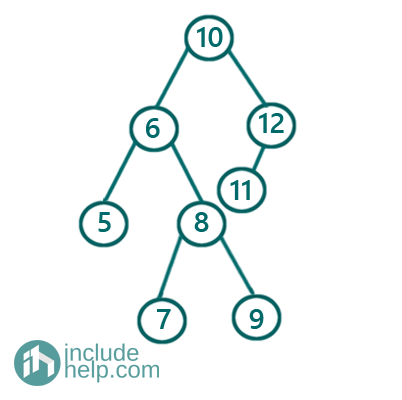Case 1: When the node to be deleted is a leaf node

Let's say we want to delete any leaf node, for example, 9 then a simple removal is enough. Below is the pictorial representation: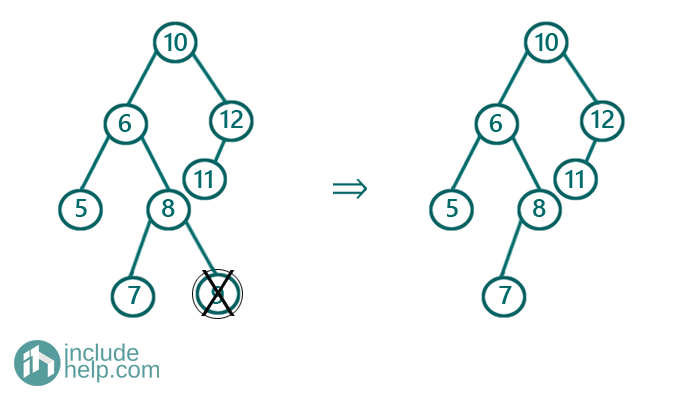Case 2: When the node to be deleted is a single child-parent

Say for the given tree we want to remove 12 which has one child 11. In this case, 11 replaces 12 since it has no more child. So the rule for this case, we can replace the node to be deleted with its child( child can be a subtree in fact). Below is the pictorial representation: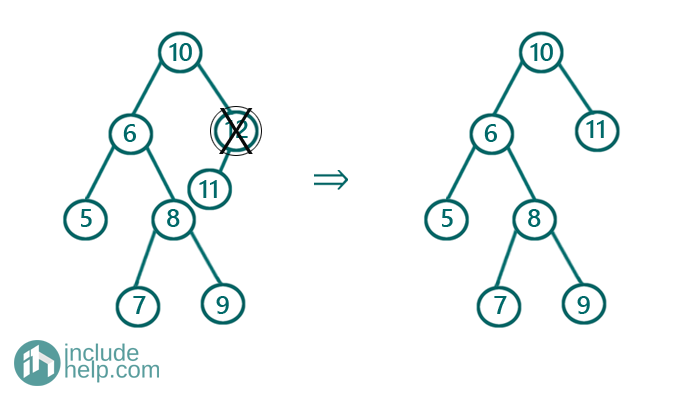Case3: When the node to be deleted is a full node

This is the most important case where a simple removal may break the BST. In this case, to ensure that even after deletion the resulting tree remains a BST, we need to follow a procedure to delete the tree. The idea is to swap the node(to be deleted) value with its inorder successor(leftmost node in the right subtree) and to delete that inorder successor node which is a leaf node and we can do simple removal. Below is the pictorial representation: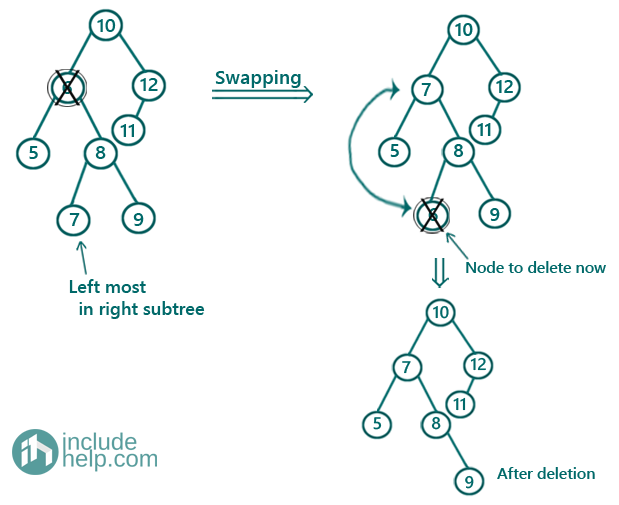C++ Implementation:

```#include <bits/stdc++.h>
using namespace std;

// tree node is defined
class TreeNode {
public:
int val;
TreeNode* left;
TreeNode* right;
TreeNode(int data)
{
val = data;
left = NULL;
right = NULL;
}
};

void inorder(TreeNode* root)
{
if (!root)
return;
inorder(root->left);
cout << root->val << " ";
inorder(root->right);
}

TreeNode* leftMost(TreeNode* node)
{
while (node->left)
node = node->left;
return node;
}

// Return the root of the modified BST after
//deleting the node with value X
TreeNode* deleteNode(TreeNode* root, int key)
{
if (!root)
return NULL;

if (root->val < key)
root->right = deleteNode(root->right, key);
else if (root->val > key)
root->left = deleteNode(root->left, key);
else {
if (!root->left)
return root->right;
else if (!root->right)
return root->left;

//root has both its children
//find the inorder successor
TreeNode* inorderSuccessor = leftMost(root->right);
//swap values
int temp = root->val;
root->val = inorderSuccessor->val;
inorderSuccessor->val = temp;
//delete the swapped
root->right = deleteNode(root->right, temp);
}

return root;
}

int main()
{
//building the tree like the example
TreeNode* root = new TreeNode(10);

root->left = new TreeNode(6);
root->right = new TreeNode(12);
root->left->left = new TreeNode(5);
root->left->right = new TreeNode(8);
root->right->left = new TreeNode(11);
root->left->right->left = new TreeNode(7);
root->left->right->right = new TreeNode(9);

cout << "Deleting node 6...\n";
root = deleteNode(root, 6);
cout << "Deletion completed...\n";
cout << "Inorder traversal after deletion\n";
inorder(root);
cout << endl;

cout << "Deleting node 12...\n";
root = deleteNode(root, 12);
cout << "Deletion completed...\n";
cout << "Inorder traversal after deletion\n";
inorder(root);
cout << endl;

cout << "Deleting node 9...\n";
root = deleteNode(root, 9);
cout << "Deletion completed...\n";
cout << "Inorder traversal after deletion\n";
inorder(root);
cout << endl;

return 0;
}
```

Output:

```Deleting node 6...
Deletion completed...
Inorder traversal after deletion
5 7 8 9 10 11 12
Deleting node 12...
Deletion completed...
Inorder traversal after deletion
5 7 8 9 10 11
Deleting node 9...
Deletion completed...
Inorder traversal after deletion
5 7 8 10 11
```

Dry Run:

So the tree is the same one as in the example.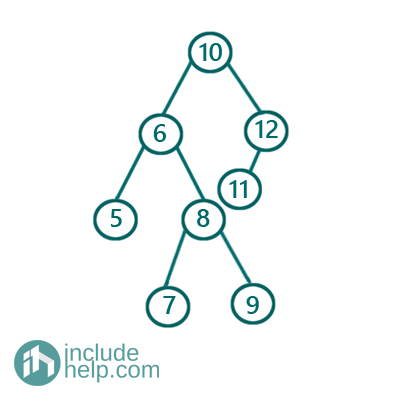Firstly, Deleting node 6

This follows the case 3 as per our above discussion. If we do the dry run then we would first search for the node 6 to delete it. Below are step by step dry run.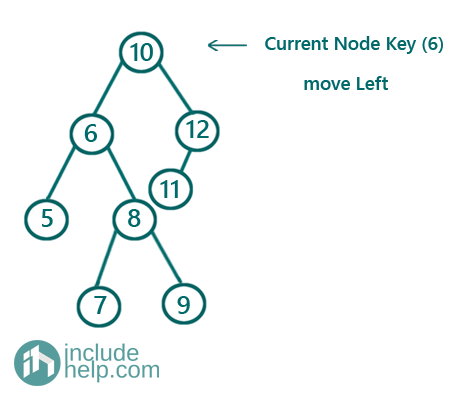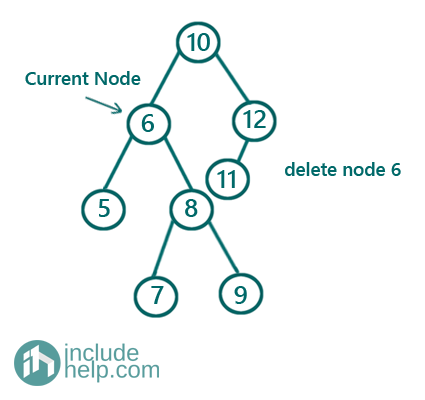As we have found the node to delete and it falls under case 3 so we will swap the node value with its inorder successor which is 7 here. And then we will delete the inorder successor which has value 6 now.Now deleting 12

Similarly, we will search for 12 first.

Now, this node has only one child and thus it falls under case 2 and above is the step to delete that.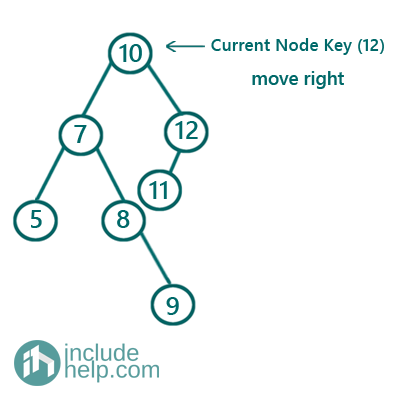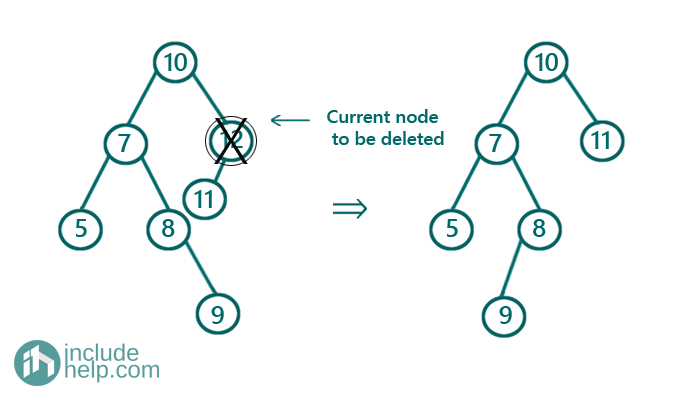Deleting 9

First search for 9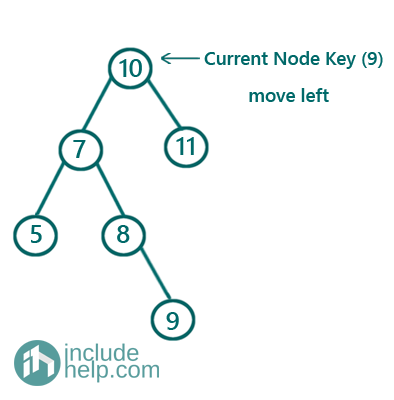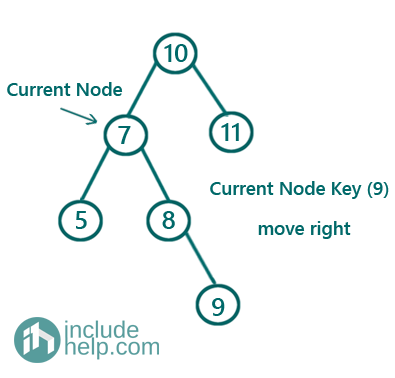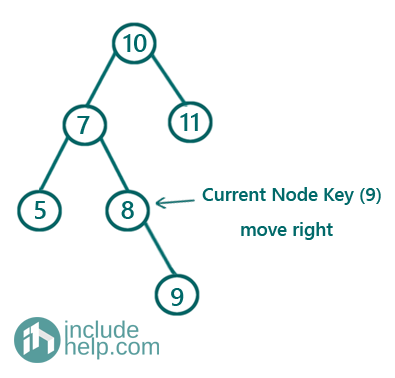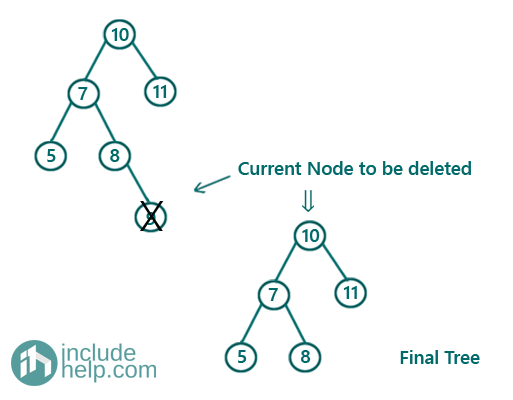As we found that the node to be deleted is a leaf node thus it falls under case 1 and simple removal is enough.

Top MCQs

Languages: » C » C++ » C++ STL » Java » Data Structure » C#.Net » Android » Kotlin » SQL
Web Technologies: » PHP » Python » JavaScript » CSS » Ajax » Node.js » Web programming/HTML
Solved programs: » C » C++ » DS » Java » C#
Aptitude que. & ans.: » C » C++ » Java » DBMS
Interview que. & ans.: » C » Embedded C » Java » SEO » HR
CS Subjects: » CS Basics » O.S. » Networks » DBMS » Embedded Systems » Cloud Computing
» Machine learning » CS Organizations » Linux » DOS
More: » Articles » Puzzles » News/Updates